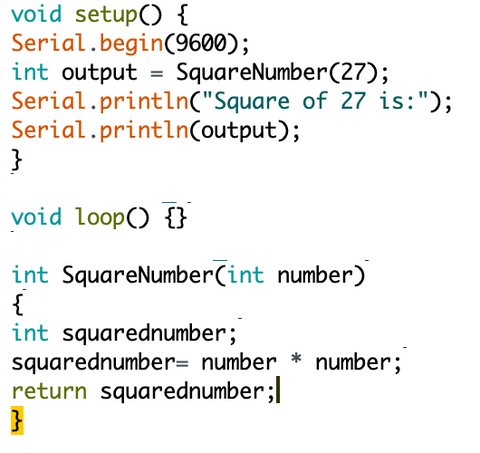translation missing: en.general.accessibility.skip_to_content

Using a Function When Programming an Arduino

What is a function

A function is a section of code that's designed to perform a specific action or task. It's useful when you need to perform this action/task multiple times in your program as a function only uses one line of code. This helps to keep your program organised, short, and simple to understand. As each time the function is "called" it uses the same lines of code it eliminates any potential errors from rewriting or copying code. You may not know but each Arduino sketch you write already uses two functions, the setup() and loop() functions. In this guide we'll be looking at the parts needed to create a function and also a some examples of how they can be used. We're using an Arduino Uno but the same applies to any Arduino based board.

Structure of a Function

Return type function name ( argument 1, argument 2, argument 3, ...)

{

Statements

}

• Return Type: This is the data type of the value returned by the function (int, float, ect). If no value if returned Void can be used.
• Function Name: This is the text you'll use when calling the function, it's worth naming it something that would help explain it's function. It can contains any letters (A-Z) and numbers (0-9). A function can not be named the same as a reserved keyword or other function.
• Arguments: These are the data values passed into the function when it is called that are required by the statements in the function.
• Statements: These are the line of code that perform the action of the function when it is called.

Calling a Function (Example Code)In this code we create a function which outputs a line of text. As no data is returned from the function it has a type of void and no arguments are passed when it is called. You can see the output below of the serial monitor where the text from the function is outputted.Calling a Function with an ArgumentIn this function we declare that it will return an integer and also uses an integer as an argument. When it is called we pass the value 27 into the function and assign the returned value to the variable output. Inside the function the argument (27) is squared and the output is returned out of the function.